• 元组的元素是不可修改的； 列表的元素可变的； 字典的元素键是不可变的，键对应的值是可变的； 集合的元素是可变的。 2、创建空的方式不同 创建空元组： tup = (); 创建空列表： list = []; 创建空集合： sets = ...
1、元素是否可修改
元组的元素是不可修改的；列表的元素可变的；字典的元素键是不可变的，键对应的值是可变的；集合的元素是可变的。
2、创建空的方式不同 创建空元组：
tup = ();

创建空列表：
list = [];

创建空集合：
sets = set();

创建空字典：
dict = { };

展开全文python
• tuple: 用圆括号（）表示，元组的特点是只读，保存在里面的元素不能修改，在保存不...Python将不能修改的值称为不可变的，而不可变的列表被称为元组。相比于列表元组是更简单的数据结构。如果需要存储的一组值在程...
tuple: 用圆括号（）表示，元组的特点是只读，保存在里面的元素不能修改，在保存不可改变的元素时，非常有优势。也有说元组是只读list。列表非常适合用于存储在程序运行期间可能变化的数据集。列表是可以修改的，然而，有时候你需要创建一系列不可修改的元素，元组可以满足这种需求。Python将不能修改的值称为不可变的，而不可变的列表被称为元组。相比于列表，元组是更简单的数据结构。如果需要存储的一组值在程序的整个生命周期内都不变，可使用元组。
list：用方括号[]表示
dict：用大括号表示
元组使用的特殊方法：
In : a = {'id':10065}
In : b = [1, 2 , 3 , 4, 5]
In : cache = (a, b)
In : (d,e) = cache #取出元组中保存的变量，将元组中保存的东西赋值给d，e两个变量，结果是a赋给d，b赋给了e
In : print(d)
{'id': 10065}
In : print(e)
[1, 2, 3, 4, 5]
In : (f) = cache #将元组中的东西赋给了f这一个变量，将全部内容赋值给了f
In : print(f)
({'id': 10065}, [1, 2, 3, 4, 5])
In : (h,i,j) = cache #将元组中的元素，赋值给三个元素的话，会提示值不够了。
---------------------------------------------------------------------------
ValueError Traceback (most recent call last)
in ()
----> 1 (h,i,j) = cache
ValueError: not enough values to unpack (expected 3, got 2)
神经网络编程中元组的使用示例：
def forward_propagation(X, parameters):
"""
Implements the forward propagation (and computes the loss) presented in Figure 2.
Arguments:
X -- input dataset, of shape (input size, number of examples)
parameters -- python dictionary containing your parameters "W1", "b1", "W2", "b2", "W3", "b3":
W1 -- weight matrix of shape ()
b1 -- bias vector of shape ()
W2 -- weight matrix of shape ()
b2 -- bias vector of shape ()
W3 -- weight matrix of shape ()
b3 -- bias vector of shape ()
Returns:
loss -- the loss function (vanilla logistic loss)
"""
# retrieve parameters
W1 = parameters["W1"]
b1 = parameters["b1"]
W2 = parameters["W2"]
b2 = parameters["b2"]
W3 = parameters["W3"]
b3 = parameters["b3"]
# LINEAR -> RELU -> LINEAR -> RELU -> LINEAR -> SIGMOID
Z1 = np.dot(W1, X) + b1
A1 = relu(Z1)
Z2 = np.dot(W2, A1) + b2
A2 = relu(Z2)
Z3 = np.dot(W3, A2) + b3
A3 = sigmoid(Z3)
cache = (Z1, A1, W1, b1, Z2, A2, W2, b2, Z3, A3, W3, b3) //将所有前向传播过程中的参数计算出来之后，保存到元组cache中。这些值在元组中不可更改
return A3, cache
def backward_propagation(X, Y, cache):
"""
Implement the backward propagation presented in figure 2.
Arguments:
X -- input dataset, of shape (input size, number of examples)
Y -- true "label" vector (containing 0 if cat, 1 if non-cat)
cache -- cache output from forward_propagation()
Returns:
gradients -- A dictionary with the gradients with respect to each parameter, activation and pre-activation variables
"""
m = X.shape
(Z1, A1, W1, b1, Z2, A2, W2, b2, Z3, A3, W3, b3) = cache #在反向传播过程中将元组中#保存的结果取出来，然后使用这些值，计算梯度。
dZ3 = A3 - Y
dW3 = 1./m * np.dot(dZ3, A2.T)
db3 = 1./m * np.sum(dZ3, axis=1, keepdims = True)
dA2 = np.dot(W3.T, dZ3)
dZ2 = np.multiply(dA2, np.int64(A2 > 0))
dW2 = 1./m * np.dot(dZ2, A1.T)
db2 = 1./m * np.sum(dZ2, axis=1, keepdims = True)
dA1 = np.dot(W2.T, dZ2)
dZ1 = np.multiply(dA1, np.int64(A1 > 0))
dW1 = 1./m * np.dot(dZ1, X.T)
db1 = 1./m * np.sum(dZ1, axis=1, keepdims = True)
gradients = {"dZ3": dZ3, "dW3": dW3, "db3": db3,
"dA2": dA2, "dZ2": dZ2, "dW2": dW2, "db2": db2,
"dA1": dA1, "dZ1": dZ1, "dW1": dW1, "db1": db1}
return gradients
展开全文• 元组（tuple）元组的创建通过赋值的方法创建元组定义元组时必须在元组后加逗号通过工厂的方法创建元组元组的索引切片连接重复成员操作符（常用来判断语句）元组的循环（元组是一个可迭代对象）test : 模拟端口扫描器...
元组（tuple）
元组的创建
通过赋值的方法创建元组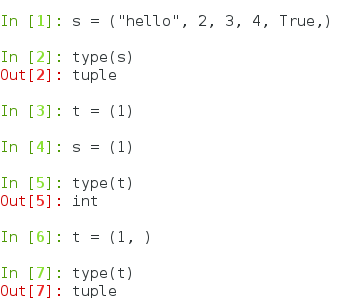定义元组时必须在元组后加逗号
通过工厂的方法创建元组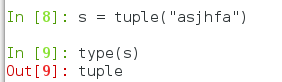元组的索引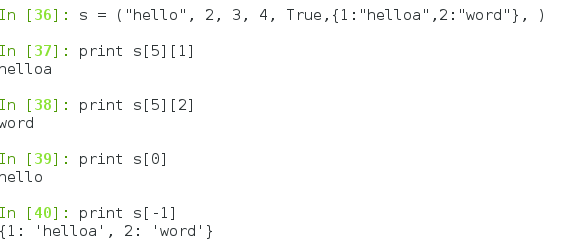切片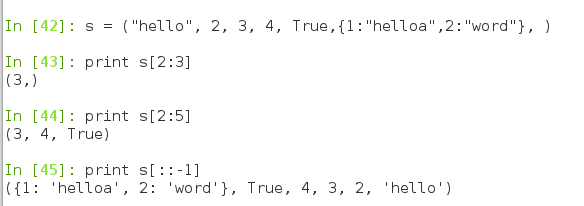连接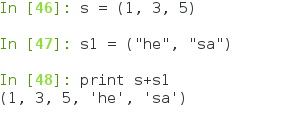重复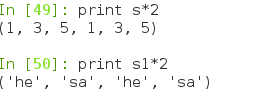成员操作符（常用来判断语句）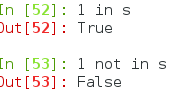元组的循环（元组是一个可迭代对象）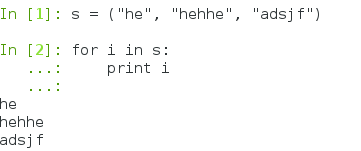test : 模拟端口扫描器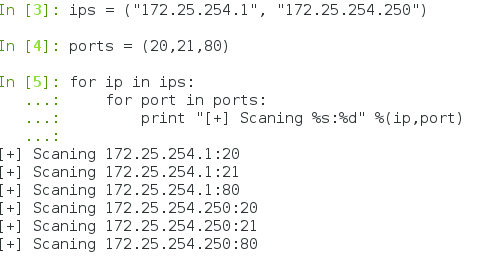元组的常用方法
count()    括号里是元组的value，返回这个value出现的次数，若是改value不在这个元组内则返回0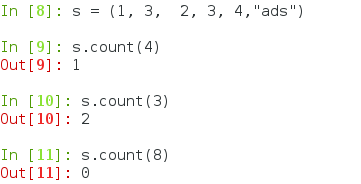.index() 方法
str.index（）
str    指定检索的字符串    beg    开始索引，默认为0    end     结束索引，默认为字符串的长度；如果不包含字符串的索引则报错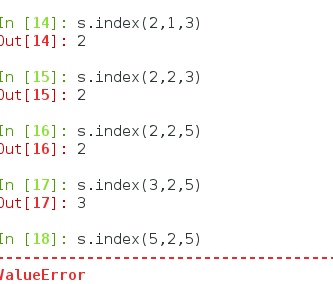元组可用的内置方法
cmp()
两个元组间第一个元素ASCII码的比较，左边大余右边的元组，返回1，左边小于右边的元组，则返回-1
若是左右两边元组相等，则返回0
max() 返回的是值大的元组    min() 返回的是值小的元组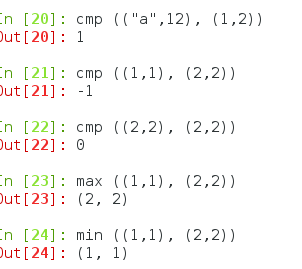枚举的使用     ： 采用元组套元组的方式
zip()    使元组的a元素和元组的b元素一一对应，若是两个元组的元数不等，则以元素少的为标准，一一匹配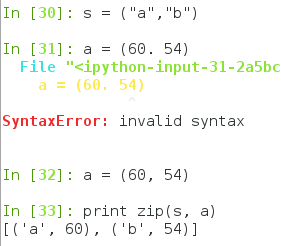https://mp.csdn.net/postedit/79631232
列表（list）
列表是可以储存多种数据类型的结构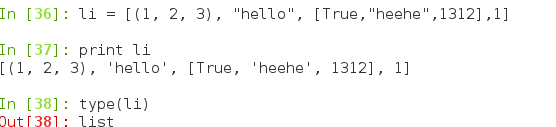列表的操作（和元组比较）
索引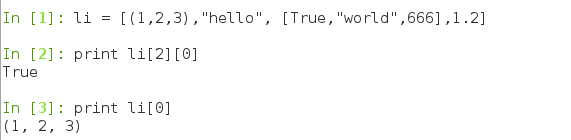列表是可变数据类型，可以修改元素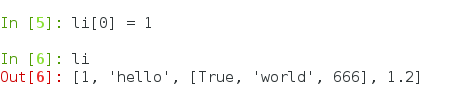元组是不可改变的数据类型，不可以修改元素
列表的切片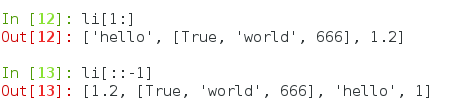重复 和连接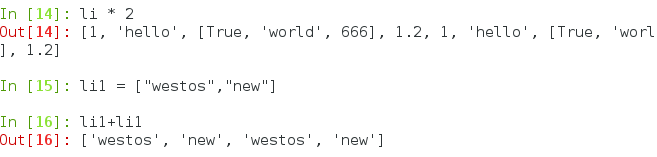成员操作符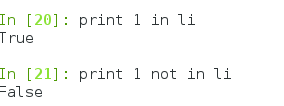列表的增删改查
增加(增加到元素列表最后，指定位置，多个元素)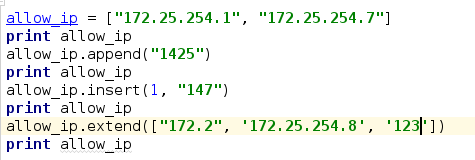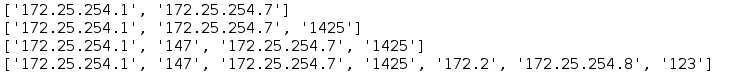删除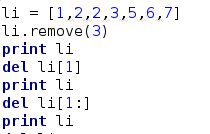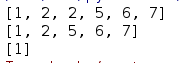如果想直接删除的话就直接删除表名就行了 del li
li.pop()    删除列表中第一个索引
修改    通过索引值进行修改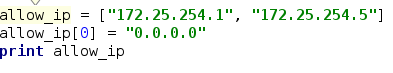查看    查看改元素所在列表的索引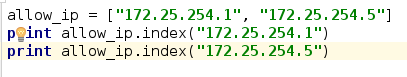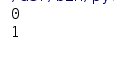stack = []info = """            栈操作                            1. 入栈    2. 出栈    3. 栈长度    4. 栈顶元素    5. 退出"""print infowhile 1:    choice = raw_input('清输入你的选择:')    if choice == '1':        in_value = raw_input('入栈元素:')        stack.append(in_value)        print  "元素%s入栈成功!" % (in_value)        print stack    elif choice == '2':        # if stack != []:   判断列表是否为空；        if stack:            out_value = stack.pop()            print "%s 出栈成功!" %(out_value)            print stack        else:            print "栈为空!"    elif choice == '3':        print "栈长度为%d" %(len(stack))    elif choice == '4':        # if stack != []:   判断列表是否为空；        if stack:            print "栈顶元素为: %s" %(stack[-1])        else:            print "栈为空!"    elif choice == '5':        exit(0)    else:
print "请输入正确的选择!"

展开全文• 首先，简单介绍一下： 元组：() 列表：[] 集合：{} 字典：{key，value}元组，一旦定义了就是不可变的 ... print tuple //读取元素列表，与数组类似，与元组区别就是可以修改 eg:list = [1,2,3] list.appen
首先，简单介绍一下：  元组：()  列表：[]  集合：{}  字典：{key，value}
元组，一旦定义了就是不可变的  eg:
    tuple = (1,2,3)
print tuple.index(1)  //查找1的位置
print tuple //读取元素
列表，与数组类似，与元组的区别就是可以修改  eg:
list = [1,2,3]
list.append(4)//在末尾追加一个4
list.pop(1)//删除第二个
list.insert(1,2)//在第二位置添加一个2
集合，和数学中的概念相似，不能重复的元素，有可变与不可变的区分  eg:
    setnor = {1,2,3}
print setnor

tuple = (1,2,3)
setfro = frozenset(tuple)
print setfro//frozenset([1, 2, 3])

settup = set(tuple)
print settup//

字典，相当于Hashtable，保存键值对。
    dict = {'name':'xiaoming','sex':'man'}
print dict//{'name': 'xiaoming', 'sex': 'man'}

print dict['name']//xiaoming

dict.setdefault('friends',{})='xiaohua'
print dict//{'friends': {1: 'xiaohua'}, 'name': 'xiaoming', 'sex': 'man'}
定义一个字典的嵌套，friends的值对应与又一个字典  注：字典的键不能重复，不会报错，但会覆盖
展开全文python
• # 3.1 数据结构序列image.png元组元组 tup 是一种固定长度、不可变的 Python 对象序列。创建元组最简单的办法就是用逗号分隔序列值元组不可修改tuple() # 任意序列或迭代器转换为元组+ 来连接元组来生成更长的元组...
• Python中,有3种内建的数据结构:列表元组和字典。 1.列表  list是处理一组有序项目的数据结构，即你可以在一个列表中存储一个序列的项目。列表中的项目。列表中的项目应该包括在方括号中，这样python就知道你是...
• Imagine a social network website which allows people to specify which other people they like.We can store information about who likes who in a list of tuples, such as the one assigned tofriendface bel...
• 列表元组、集合、字典区别列表元组集合字典英文listtuplesetdict可否读写读写只读读写读写可否重复是是否是存储方式值值键(不能重复)键值对(键不能重复)是否有序有序有序无序无序，自动正序初始化[1,'a']('a', 1...
• 主要介绍了python元组列表字典，文中通过示例代码介绍的非常详细，对大家的学习或者工作具有一定的参考学习价值，需要的朋友们下面随着小编来一起学习学习吧
• 元组——tuple：和列表功能相似，但不能改变其元素的值，即不可以进行增删改的操作，只能执行查询操作。用小括号表示(num1,num2,num3) 字典——infor：类型不同的元素，由键值对组成，与列表一样可以进行增删改查。...
• 最近几天在学python的四种结构化类型，分别是元组(tuple) 列表(list) 范围(range) 字典(dict)，其中元组相对简单，它是字符串的扩展，它长度不可变的有序序列,列表是长度可变的有序序列，范围是不可变的，字典和列表...
• python学习笔记01-列表元组和字典区别 前言： python学习中，经常会用到列表元组和字典，对于新学者而言，往往不能清晰的了解分辨三者之前的具体差别。为了解决这一点，方便后续复习，特总结本篇文章，作为...python
• 列表元组和字典 1. 通用序列操作 Pyhton中有六种内置序列：列表元组、字符串、Unicode字符串、buffer对象xrange对象，其中列表元组和字符串这三种序列是最常见的。 Python中的大部分序列都可以进行通用操作...python 集合
• 本文主要介绍列表元组字典，集合以及字符串之间的相互转换。1. 列表元组的互换# 将列表转化为元组li = [1, 2, 3]t = tuple(li)print(t, type(t))# 打印结果:(1, 2, 3) # 将元组转换成列表tu = (1, 2, 3)li =...
• 我有一个偶数长度的元组,其元素如(‘a’,’b’,’c’,’d’,’e’,’f’)我想转换为字典,其元素如[‘a’：’ b’,’c’：’d’,’e’：’f’].我尝试使用dict(元组),但这没有帮助.我刚开始学习Python,任何帮助都会...
• 目录标题 一、从列表中取出字典的keyvalue二、取出列表元组中的字典 一、从列表中取出字典的keyvalue 例: list_1=[{'name':'张三'},{'age':12},{'sex':'女'}] for i in list_1: for key,value in i.items(): ...python
• 列表 元组 字典 列表 list是一种有序的数据集合，可以随意的添加删除其中的数据，用[]表示 可以使用索引来获取列表中的元素，索引是从0开始的 列表常用命令 获取列表长度：len() 添加元素：append() 在指定位置...
• 理解Python中列表元组字典，集合的区别 列表元组字典，集合的区别是python面试中最常见的一个问题。这个问题虽然很基础，但确实能反映出面试者的基础水平。 1.列表（List） 什么是列表呢？我觉得列表就是...
• 1、列表：专门用于存储一串信息,列表用中括号[]定义,数据之间使用逗号,分隔list_0=['a','b','c']列表的特点：1）可修改、可增加、可删除2）列表是有序的，可以遍历3）可以将元组转化为列表、字符串转化为列表，如下...
• 名称 英文 使用符号 是否可修改 元组 tuple 小括号 () 否 列表 list 中括号 [] 是 字典 dict 大括号 {} 是list python dict
• 列表,元组和字典区别 列表的定义：列表可以有序的存储任何数据类型。 格式：列表名=[ ] 列表是有序的数据类型可以进行（索引.切片） 索引有：in not in index count 切片：用来获取字符串中的某个字符或是某一段...python
• 列表 元组 集合 字典 英文 list tuple set dict 定义 定义[],数据之间使用,分割 定义()，数据之间用,分割 定义用 {} 或 set() 字典的每个键值 key=&gt;value 对用冒号 ...
• python的3种内建数据结构: 元组（tuple）、列表（list）、字典（dictionary） 一、元组（tuple） 元组是有序的不可变序列。元组不能被修改。通过圆括号（）中用逗号，分隔的项目定义。可以通过序号访问（t[index])。...
• 列表和元组是Python中最常用的两种数据结构，字典是第三种。 相同点：都是序列都可以存储任何数据类型可以通过索引访问图片.png语法差异使用方括号[]创建列表，而使用括号()创建元组。 请看以下示例：>>>l=[...
• Python列表,元组,字典区别： 1、元组是不可变的， 而列表字典是可变的。元组是不可变对象，对象一旦生成，它的值将不能更改；列表是可变对象，对象生成后，可以对其元素进行更改、添加、删除、清空、排序等...
• 一、Python中的列表元组和字典 1、介绍： Python中最为常用的数据容器有列表元组和字典。是使用Python必须掌握的基础。本文详细介绍列表元组和字典，以及字典的两种（按照key排序按照value排序）排序方法。 二...python
• 3.5将二维结构列表和元组转换成字典 l = [["a", 1], ["b", 2]] t = (("x", 3), ("y", 4)) d_l = dict(l) d_t = dict(t) print(d_l) print(d_t) 结果为 {'a': 1, 'b': 2} {'x': 3, 'y': 4}
• 元组和字典 元组 1.什么是元组 元组是不可变的列表 元组也是容器型数据类型，将（）作为容器标志多个元组之间用逗号隔开：（元素1，元素2，元素3.。。。） 元组不可变（不能增删改）；元组是有序的（支持下标操作） ...python...